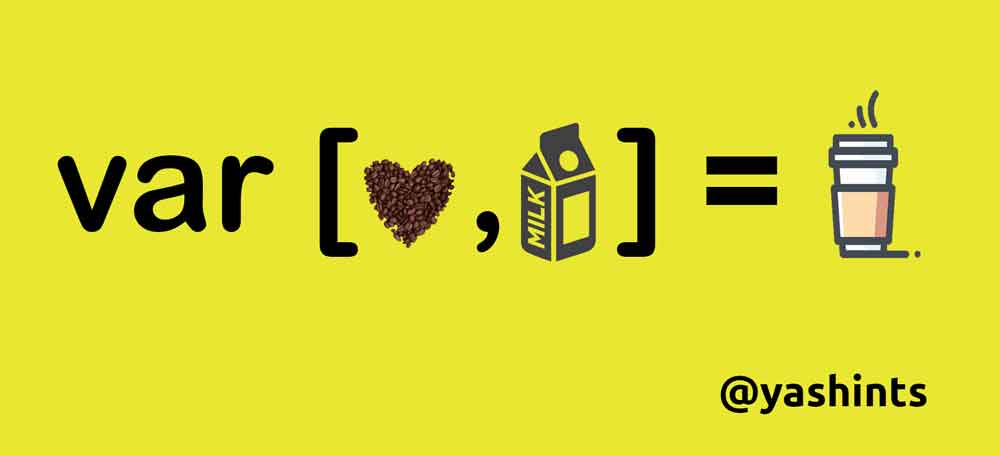Did you know that you can destructure arrays in PHP, just like you can in ES6 JavaScript?

Destructuring allows us to unpack the values from an array. So if you had code that returned an array of values then you could assign those values to variables immediately.

So, let’s see the difference. An explode function will separate a string into an array by a passed character.

``````\$array = explode('|', 'one|two');

echo \$array; // one
echo \$array; // two
``````

However, with array destructuring we can get the values from the function and assign it to variables right away. For example:

``````[\$one, \$two] = explode('|', 'one|two');

echo \$one; // one
echo \$two; // two
``````

Now, we can assign the values right away. How cool is that!

### EXTRA BONUS HIDDEN FEATURE TIME!

Now for something really cool. What if we want to skip a variable? Well, we can do this! All you need is a comma! In the following example we will get the first and third, but skip the second because we don’t need it (for whatever reason).

``````[\$one, , \$three] = explode('|', 'one|two|three');

echo \$one; // one
echo \$three; // three
``````

All we did was skip it. You can use the comma to skip variable assignments in array destructuring as much as you want!

``````[\$one, , , \$four] = explode('|', 'one|two|three|four');

echo \$one; // one
echo \$four; // four
``````